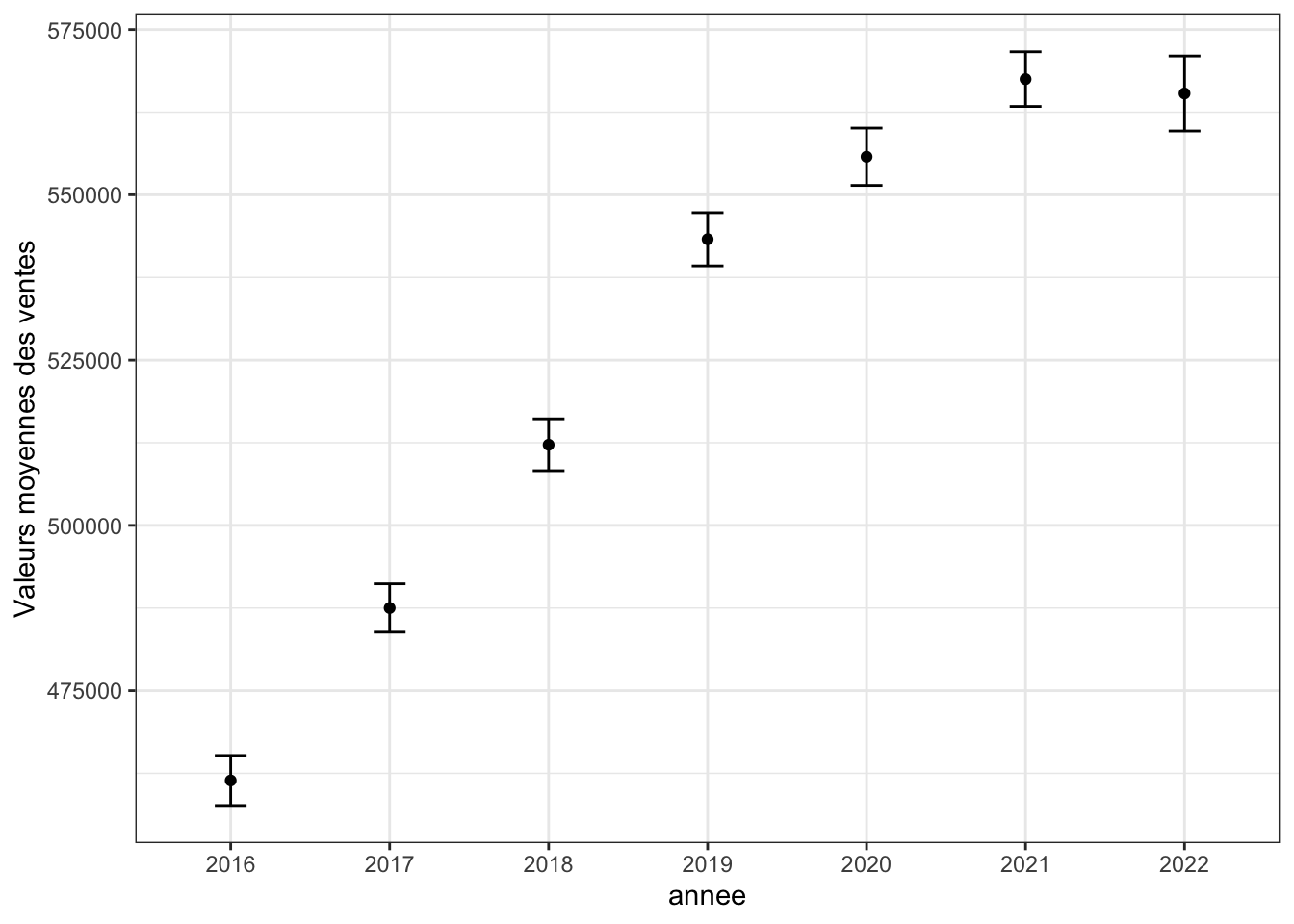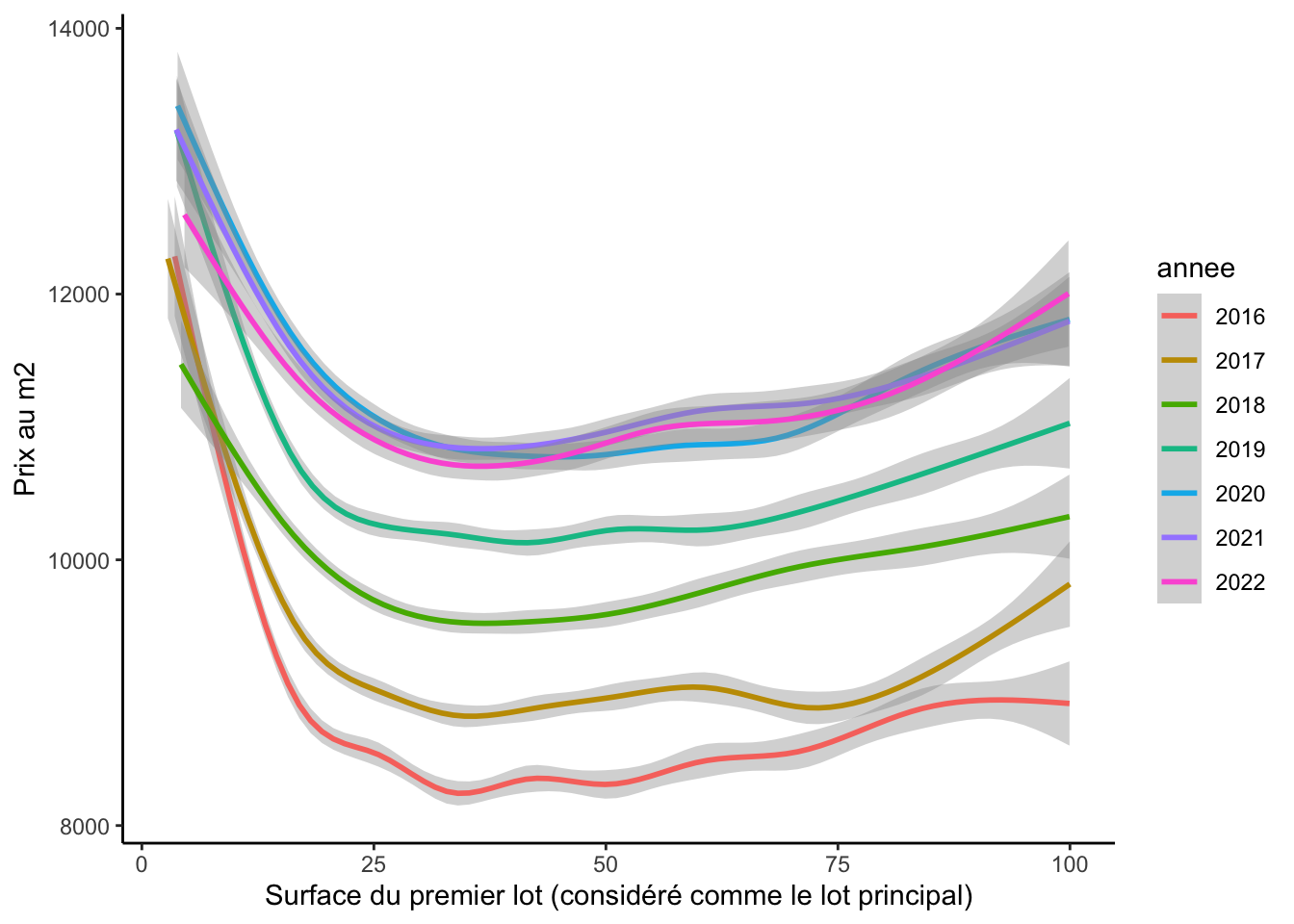# 1 The parisian house market and `data.gouv.fr`

## 1.1 Question 1

This plot is a bit complicated, in particular the confidence intervals.

The different steps are:

• stack the dataframes with `rbind`

• filter a subtable without extreme sales and without the row having missing values in `valeur_fonciere`

• create a colunm year, for this you can use the package `lubridate`, but you can also do it alone with a package extracting the first four characters of a string.

• then use the function `group_by`, to group by year the observations and compute the mean and the standard deviation

• then plot the average over years

• the 95% confidence intervals are obtained from the standard deviation

``````knitr::opts_chunk\$set(echo = TRUE)

# We could have done a loop to avoid copy paste, but let's keep it like this for the first class
# Be careful to put the right path (here, I put all the raw data in a folder named 'data')

immo <- rbind(df_2016, df_2017, df_2018, df_2019, df_2020, df_2021, df_2022)

# at the beginning of your notebook
VALEUR_FONCIERE_MAX = 2000000
VALEUR_FONCIERE_MIN = 50000

# Libraries used
library(ggplot2)
library(dplyr) # group_by
library(lubridate) # function to treat automatically year

# Data treatment

## Only keep costal code from Paris
df <- immo[immo\$code_postal %in% c( paste0("7500",1:9),paste0("750",10:20)) ,]

## Define which columns we want to keep
useful_columns <- c("date_mutation",
"valeur_fonciere",
"nature_mutation",
"code_postal",
"nombre_pieces_principales",
#"surface_reelle_bati",
"lot1_surface_carrez",
"type_local",
"nom_commune",
"lot2_surface_carrez",

## take subtable
immo <- immo[immo\$valeur_fonciere < VALEUR_FONCIERE_MAX,]
immo <- immo[immo\$valeur_fonciere > VALEUR_FONCIERE_MIN,]

## create a column year
immo\$annee <- year(immo\$date_mutation)

## remove duplicated lines
nrow(df)``````
``##  441122``
``````df <- unique(df)
nrow(df)``````
``##  413128``
``````## function from package lubridate to get the year and month
immo\$month <- month(immo\$date_mutation, label=TRUE, abbr=FALSE)
immo\$annee <- as.factor(immo\$annee)
# An alternative could be to use `gsub`

# Only keep housing
immo <- immo[immo\$type_local %in% c("Appartement", "Maison"),]

# Only keep transactions
immo <- immo[immo\$nature_mutation == "Vente",]

# Keep only observations for which valeur fonciere is observed
immo <- immo[!is.na(immo\$valeur_fonciere),] # ! indicates "opposite to" so that we keep only rows for which "valeur_fonciere" is observed``````

Check how many transaction by year. Does it seem reasonnable?

``table(immo\$annee)``
``````##
##  2016  2017  2018  2019  2020  2021  2022
## 32541 37086 35264 35854 29401 33933 17979``````
``````summary <- immo  %>%
group_by(annee) %>%
summarise(mean = mean(valeur_fonciere),
sd = sd(valeur_fonciere),
n = n())

summary\$conf.int.inf = summary\$mean - 1.96*summary\$sd / sqrt(summary\$n)
summary\$conf.int.sup = summary\$mean + 1.96*summary\$sd / sqrt(summary\$n)

ggplot(summary, aes(x = annee, y = mean)) +
geom_point()+
geom_errorbar(aes(ymin=conf.int.inf, ymax=conf.int.sup), width=.2,
position=position_dodge(0.05)) +
theme_bw() +
ylab("Valeurs moyennes des ventes")``````💡 In reality, those confidence intervals do not really have an interest as we have access to the whole data set. A confidence intervals reflects the uncertainty, and more precisely the fact that you have sampled a part of an infinite (or way bigger) population. This is clearly not the case here as we have access to the whole transactions. But this is for you to practice.

## 1.2 Question 2

First, we need to do other assumptions

``````## only keep element with surface
immo <- immo[!is.na(immo\$lot1_surface_carrez),]

## Create price per squarre meters: assumptions here on the fact that the lot 1 is the principal one.
immo\$prix.m2 <- immo\$valeur_fonciere / immo\$lot1_surface_carrez``````
``````ggplot(immo[immo\$prix.m2 < 30000 & immo\$lot1_surface_carrez < 100,], aes(x = lot1_surface_carrez, y = prix.m2, group = annee, color = annee)) +
geom_smooth() +
theme_classic() +
ylab("Prix au m2") +
xlab("Surface du premier lot (considéré comme le lot principal)")``````
``## `geom_smooth()` using method = 'gam' and formula = 'y ~ s(x, bs = "cs")'``## 1.3 Question 3

Then, we can observe the number of transactions per month over year to understand what happened in 2020.

``````# 2021 is only first semester so we remove it from the plot
immo[immo\$annee != 2022,] %>%
group_by(annee, month) %>%
summarise(count = n()) %>%
ggplot(aes(x = month, y = count, color = as.factor(annee), group = as.factor(annee))) +
geom_line(linewidth = 1) +
theme_classic() +
theme(axis.text.x = element_text(angle = 90, vjust = 0.5, hjust=1)) +
ylab("Nombre de transactions par mois") +
xlab("")``````
``````## `summarise()` has grouped output by 'annee'. You can override using the
## `.groups` argument.``````